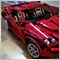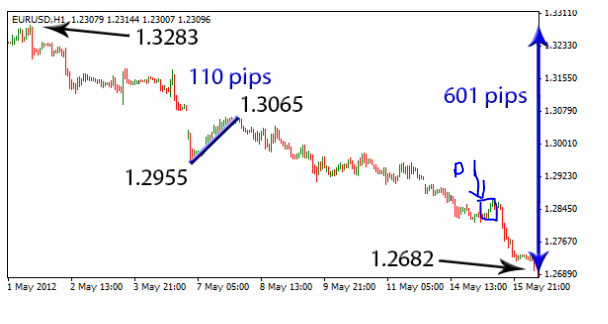# Calculating max drawdown in xx candles help844

Does anyone know how to perform the max drawdons in xx candles?

I have an example of symbol prices like below:

 Candle Close high Diference 0 1.258 1.265 0.007 1 1.265 1.274 0.009 2 1.274 1.286 0.012 3 1.286 1.3 0.014 4 1.3 1.29 -0.01 5 1.29 1.291 0.001 6 1.286 1.285 -0.001 7 1.285 1.283 -0.002 8 1.283 1.281 -0.002 9 1.281 1.29 0.009 10 1.29 1.3 0.01

This is an uptrend because the instrument increased during that period from i.e  from 1.258 to 1.29. So the maximum drawdown is the period or the sum of the candle/candles  in series or  the candle with the biggest loss ; sums of candles 6, 7 and 8 = -0.05.

so max drawdown is -0.05

How do  calculate this max drawdown in mql5?

No problem in the calculation of the difference between the close and high but have difficulties in summing up the consecutive losses, is there a special function that can perform this in Mql5?425

Peter Kaiza:

No problem in the calculation of the difference between the close and high but have difficulties in summing up the consecutive losses, is there a special function that can perform this in Mql5?

If I understand you question correctly, here's your 'special function' :

```double CalcMaxDD(int xx)
{
double maxdd = 0;
for (int i=1; i<=xx; i++)
{
double diff = high[i]-close[i];
if (diff < 0)
maxdd = maxdd + diff;
}
return (maxdd);
}```
But it's strange though... under what condition(s) will the high price of a candle be higher than its close price?844

Seng Joo Thio:

If I understand you question correctly, here's your 'special function' :

But it's strange though... under what condition(s) will the high price of a candle be higher OK than its close price?
That was a mistake on the close. Thanks for the function. Basically if the price is going up during the xx candles and sometime during its rise it retraces let's say 10 pips then it increases let's say 5 pips and then it  decreases again 15 pips then continues to rise. Will the function be able to calculate the maximum drawdown during those xx candles which in this example is 15,pips?
Thanks425

Peter Kaiza:

That was a mistake on the close. Thanks for the function. Basically if the price is going up during the xx candles and sometime during its rise it retraces let's say 10 pips then it increases let's say 5 pips and then it  decreases again 15 pips then continues to rise. Will the function be able to calculate the maximum drawdown during those xx candles which in this example is 15,pips?

Well if it retraces by 10, increases by 5 before retracing by another 15 then the total drawdown could either be 20 or 15, depending on where u base your 5 pips increase on (i.e from the higher of the previous high, or from the 10 pips low).

From your description basically you want the maximum drawdown from the highest float attained - in which case drawdown may not be the right term to use... I would understand it as just a price pullback. But regardless, is there a need to calculate such figures based on historical data? (as in computing it given xx bars, as opposed to monitoring/collecting such data as new ticks arrive).844Here is an example. Overall eurusd decreased from 1 May to 15 May. During that time there were periods where the price retraced up. The biggest re-tracement up is when the price moved from 1.2955 to 1.3065 ( 110 pips)  this is what i'm looking for.  You can see from the chart, another  re-trancement up was 14 May (marked D with arrow) but this is smaller than the one I mentioned and there are more others. So my question is how do you find this max retracement in  xx bars. You can see that the re-tracement was calculated from the Low of that bar to the high of the other bar( 1.3065).

My mistake, you don't  use close and open prices but   highs and lows. The technical problem for me is  the folmula has to first calculate all the retracements and deduce the highest one from these.

This is what I'm looking for. Sorry for the  previous confusion.425

Peter Kaiza:

Here is an example. Overall eurusd decreased from 1 May to 15 May. During that time there were periods where the price retraced up. The biggest re-tracement up is when the price moved from 1.2955 to 1.3065 ( 110 pips)  this is what i'm looking for.  You can see from the chart, another  re-trancement up was 14 May (marked D with arrow) but this is smaller than the one I mentioned and there are more others. So my question is how do you find this max retracement in  xx bars. You can see that the re-tracement was calculated from the Low of that bar to the high of the other bar( 1.3065).

My mistake, you don't  use close and open prices but   highs and lows. The technical problem for me is  the folmula has to first calculate all the retracements and deduce the highest one from these.

This is what I'm looking for. Sorry for the  previous confusion.

Right... Ok, I've created one for up trend, see if you can modify the FindBiggestRetracement() function to also handle down trend:

```void OnStart()
{
//---
double Result = 0;

Result = FindBiggestRetracement(160,1);

Print ("Biggest Retracement = ", Result/_Point);
}

double FindBiggestRetracement(int NumBars, int Direction)
{
if (NumBars>=iBars(NULL,0)-1)
return (0); // Error - not enough bars.

int HighBars[], LowBars[];
ArrayResize(HighBars,NumBars);
ArrayResize(LowBars,NumBars);
double HHPrice = 0, LLPrice = 0;
double MaxRetracement = 0;
int ArrayIdx = 0;
bool InRetracement = false;

if (Direction>0)
{
for (int i=NumBars; i>0; i--)
{
double HPrice = iHigh(NULL,0,i);
double LPrice = iLow(NULL,0,i);

if (HPrice>HHPrice && HHPrice!=0 && InRetracement)
{
InRetracement = false;
LLPrice = 0;
double CurrRetracement = iHigh(NULL,0,HighBars[ArrayIdx])-iLow(NULL,0,LowBars[ArrayIdx]);
if (CurrRetracement>MaxRetracement || MaxRetracement==0)
MaxRetracement = CurrRetracement;
ArrayIdx++;
}

if (HPrice>HHPrice || HHPrice==0)
{
HHPrice = HPrice;
HighBars[ArrayIdx] = i;
}
else
if (LPrice<LLPrice || LLPrice==0)
{
LLPrice = LPrice;
LowBars[ArrayIdx] = i;
InRetracement = true;
}
}

if (InRetracement)
{
double CurrRetracement = iHigh(NULL,0,HighBars[ArrayIdx])-iLow(NULL,0,LowBars[ArrayIdx]);
if (CurrRetracement>MaxRetracement || MaxRetracement==0)
MaxRetracement = CurrRetracement;
}
}

return (MaxRetracement);
}```844

Thanks Seng,

A few questions:

Because of the onstart(),I get this warning:

OnInit function is useless for scripts ....... 5

I have incorporated the onstart elements into oninit..

Also where in the function itself is the reference to how many bars to look back? Is it the 160 you referenced within onstart?425

Peter Kaiza:

Also where in the function itself is the reference to how many bars to look back? Is it the 160 you referenced within onstart?

That's right, u can replace it with other numbers, or u can modify the function to take in datetime, or even read a trendline.844

Seng

I've ammended the code to read a downtrend draw down, I've included ABS to read negative values, but it does not appear to output correct figures.

What could be changed?

```  if (Direction>0)
{
for (int i=NumBars; i>0; i--)
{
double HPrice = iHigh(NULL,0,i);
double LPrice = iLow(NULL,0,i);

if (LPrice>LLPrice && LLPrice!=0 && InRetracement)
{
InRetracement = false;
LLPrice = 0;
double CurrRetracement = MathAbs(iLow(NULL,0,LowBars[ArrayIdx])-iHigh(NULL,0,HighBars[ArrayIdx]));
if (CurrRetracement>MaxRetracement || MaxRetracement==0)
MaxRetracement = CurrRetracement;
ArrayIdx++;
}

if (LPrice>LLPrice || LLPrice==0)
{
LLPrice = LPrice;
LoWBars[ArrayIdx] = i;
}
else
if (HPrice>HHPrice || HHPrice==0)
{
HHPrice = HPrice;
HighBars[ArrayIdx] = i;
InRetracement = true;
}
}

if (InRetracement)
{
double CurrRetracement = MathAbs(iLow(NULL,0,LowBars[ArrayIdx])-iHigh(NULL,0,HighBars[ArrayIdx]));
if (CurrRetracement>MaxRetracement || MaxRetracement==0)
MaxRetracement = CurrRetracement;
}
}

return (MaxRetracement);
}```425

Peter Kaiza:

Seng

I've ammended the code to read a downtrend draw down, I've included ABS to read negative values, but it does not appear to output correct figures.

What could be changed?

You're right to change Ls to Hs and Hs to Ls, but you'll also have to check their comparison - for example LPrice and LLPrice - you want LPrice < LLPrice rather than LPrice > LLPrice. The logic is that you want to capture an LPrice that is more extreme than the previously recorded extreme which is LLPrice.

And remember to change "if (Direction>0)" to "if (Direction<0)".

Also, MathAbs is not necessary, and you need not swap iHigh and iLow since what really matters is the bar number you supply... so iHigh - iLow is still correct.844

```if (Direction<0)
{
for (int i=NumBars; i>0; i--)
{
double HPrice = iHigh(NULL,0,i);
double LPrice = iLow(NULL,0,i);

if (LPrice<LLPrice && LLPrice!=0 && InRetracement)
{
InRetracement = false;
LLPrice = 0;
double CurrRetracement = MathAbs(iLow(NULL,0,LowBars[ArrayIdx])-iHigh(NULL,0,HighBars[ArrayIdx]));
if (CurrRetracement>MaxRetracement || MaxRetracement==0)
MaxRetracement = CurrRetracement;
ArrayIdx++;
}

if (LPrice<LLPrice || LLPrice==0)
{
LLPrice = LPrice;
LowBars[ArrayIdx] = i;
}
else
if (HPrice>HHPrice || HHPrice==0)// Should I also change the > direction?
{
HHPrice = HPrice;
HighBars[ArrayIdx] = i;
InRetracement = true;
}
}

if (InRetracement)
{
double CurrRetracement = MathAbs(iLow(NULL,0,LowBars[ArrayIdx])-iHigh(NULL,0,HighBars[ArrayIdx]));
if (CurrRetracement>MaxRetracement || MaxRetracement==0)
MaxRetracement = CurrRetracement;
}
}

return (MaxRetracement);```

Seng,

See above,

I've amended  (LPrice>LLPrice) , should  the (HPrice>HHPrice) sign  be amended?

Thanks.

To add comments, please log in or register